### Home > MC1 > Chapter 8 > Lesson 8.4.2 > Problem8-117

8-117.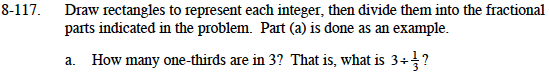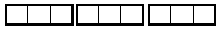As you can see, in the figure above each bold rectangle represents 1, and each 1 is divided into three parts. If you count the thirds represented, you will find find the answer to part (a).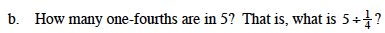Try drawing a diagram as modeled in part (a). It may help to know that there are 4 one-fourths in 1. Can you find how many are in 5?

There are 20 fourths in 5.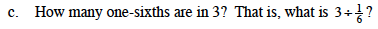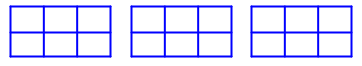Your rectangles might look something like the ones above. Can you count the one-sixths in the three rectangles?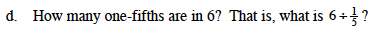The quotient is 30.